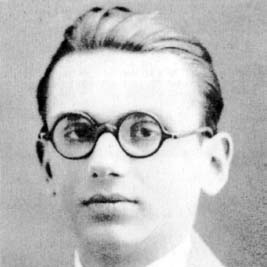NextPrevious

# What is Gödel’s incompleteness theorem?

Austrian-American mathematician and logician Kurt Gödel (1906–1978) is best known for his studies in mathematical logic—in particular for his “incompleteness theorem” presented in 1931. This theorem shows that an infinite number of propositions that cannot be derived from axioms of a system may be proved by metamathematical means external to mathematics. In other words, mathematics abounds with questions that have a “yes or no” nature; the incompleteness theory suggests that such questions will always exist. (For more about Gödel, see “History of Mathematics.”)Austrian-American mathematician and logician Kurt Gödel is best known for his studies in mathematical logic.

Close

This is a web preview of the "The Handy Math Answer Book" app. Many features only work on your mobile device. If you like what you see, we hope you will consider buying. Get the App